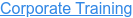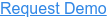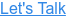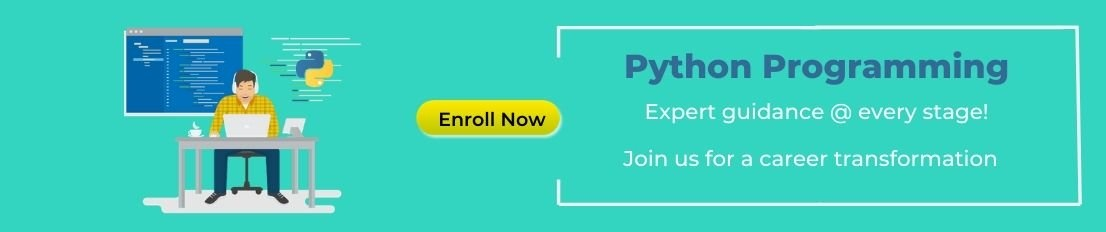#### 15+ Categories. 500+ IT Courses to Learn.# Top 25 Data Science Interview Questions and Answers

by myadmin, on May 13, 2017 1:55:50 AM## Q1. How would you create a taxonomy to identify key customer trends in unstructured data?

Ans: The best way to approach this question is to mention that it is good to check with the business owner and understand their objectives before categorizing the data. Having done this, it is always good to follow an iterative approach by pulling new data samples and improving the model accordingly by validating it for accuracy by soliciting feedback from the stakeholders of the business. This helps ensure that your model is producing actionable results and improving over the time.

## Q2. Python or R – Which one would you prefer for text analytics?

Ans: The best possible answer for this would be Python because it has Pandas library that provides easy to use data structures and high performance data analysis tools.

## Q3. Which technique is used to predict categorical responses?

Ans: Classification technique is used widely in mining for classifying data sets.

## Q4. What is logistic regression? Or State an example when you have used logistic regression recently.

Ans: Logistic Regression often referred as logit model is a technique to predict the binary outcome from a linear combination of predictor variables. For example, if you want to predict whether a particular political leader will win the election or not. In this case, the outcome of prediction is binary i.e. 0 or 1 (Win/Lose). The predictor variables here would be the amount of money spent for election campaigning of a particular candidate, the amount of time spent in campaigning, etc.

## Q5. What are Recommender Systems?

Ans: A subclass of information filtering systems that are meant to predict the preferences or ratings that a user would give to a product. Recommender systems are widely used in movies, news, research articles, products, social tags, music, etc.

## Q6. Why data cleaning plays a vital role in analysis?

Ans: Cleaning data from multiple sources to transform it into a format that data analysts or data scientists can work with is a cumbersome process because - as the number of data sources increases, the time take to clean the data increases exponentially due to the number of sources and the volume of data generated in these sources. It might take up to 80% of the time for just cleaning data making it a critical part of analysis task.

## Q7. Differentiate between univariate, bivariate and multivariate analysis.

Ans: These are descriptive statistical analysis techniques which can be differentiated based on the number of variables involved at a given point of time. For example, the pie charts of sales based on territory involve only one variable and can be referred to as univariate analysis.
If the analysis attempts to understand the difference between 2 variables at time as in a scatterplot, then it is referred to as bivariate analysis. For example, analysing the volume of sale and a spending can be considered as an example of bivariate analysis.
Analysis that deals with the study of more than two variables to understand the effect of variables on the responses is referred to as multivariate analysis.

## Q8. What do you understand by the term Normal Distribution?

Ans: Data is usually distributed in different ways with a bias to the left or to the right or it can all be jumbled up. However, there are chances that data is distributed around a central value without any bias to the left or right and reaches normal distribution in the form of a bell shaped curve. The random variables are distributed in the form of an symmetrical bell shaped curve.

Q9. What is Linear Regression?
Linear regression is a statistical technique where the score of a variable Y is predicted from the score of a second variable X. X is referred to as the predictor variable and Y as the criterion variable.

## Q10. What is Interpolation and Extrapolation?

Ans: Estimating a value from 2 known values from a list of values is Interpolation. Extrapolation is approximating a value by extending a known set of values or facts.11)       What is power analysis?
An experimental design technique for determining the effect of a given sample size.

## Q11. What is Collaborative filtering?

Ans: The process of filtering used by most of the recommender systems to find patterns or information by collaborating viewpoints, various data sources and multiple agents.

## Q12. What is the difference between Cluster and Systematic Sampling?

Ans: Cluster sampling is a technique used when it becomes difficult to study the target population spread across a wide area and simple random sampling cannot be applied. Cluster Sample is a probability sample where each sampling unit is a collection, or cluster of elements. Systematic sampling is a statistical technique where elements are selected from an ordered sampling frame. In systematic sampling, the list is progressed in a circular manner so once you reach the end of the list,it is progressed from the top again. The best example for systematic sampling is equal probability method.

## Q13. Are expected value and mean value different?

Ans: They are not different but the terms are used in different contexts. Mean is generally referred when talking about a probability distribution or sample population whereas expected value is generally referred in a random variable context.
For Sampling Data
Mean value is the only value that comes from the sampling data.
Expected Value is the mean of all the means i.e. the value that is built from multiple samples. Expected value is the population mean.
For Distributions
Mean value and Expected value are same irrespective of the distribution, under the condition that the distribution is in the same population.

## Q14. What does P-value signify about the statistical data?

Ans: P-value is used to determine the significance of results after a hypothesis test in statistics. P-value helps the readers to draw conclusions and is always between 0 and 1.

• P- Value > 0.05 denotes weak evidence against the null hypothesis which means the null hypothesis cannot be rejected.
• P-value <= 0.05 denotes strong evidence against the null hypothesis which means the null hypothesis can be rejected.
• P-value=0.05is the marginal value indicating it is possible to go either way.## Q15. Do gradient descent methods always converge to same point?

Ans: No, they do not because in some cases it reaches a local minima or a local optima point. You don’t reach the global optima point. It depends on the data and starting conditions.

## Q16. A test has a true positive rate of 100% and false positive rate of 5%. There is a population with a 1/1000 rate of having the condition the test identifies. Considering a positive test, what is the probability of having that condition?

Ans: Let’s suppose you are being tested for a disease, if you have the illness the test will end up saying you have the illness. However, if you don’t have the illness- 5% of the times the test will end up saying you have the illness and 95% of the times the test will give accurate result that you don’t have the illness. Thus there is a 5% error in case you do not have the illness.
Out of 1000 people, 1 person who has the disease will get true positive result.
Out of the remaining 999 people, 5% will also get true positive result.
Close to 50 people will get a true positive result for the disease.
This means that out of 1000 people, 51 people will be tested positive for the disease even though only one person has the illness. There is only a 2% probability of you having the disease even if your reports say that you have the disease.

## Q17. What is the difference between Supervised Learning an Unsupervised Learning?

Ans: If an algorithm learns something from the training data so that the knowledge can be applied to the test data, then it is referred to as Supervised Learning. Classification is an example for Supervised Learning. If the algorithm does not learn anything beforehand because there is no response variable or any training data, then it is referred to as unsupervised learning. Clustering is an example for unsupervised learning.

## Q18. What is the goal of A/B Testing?

Ans: It is a statistical hypothesis testing for randomized experiment with two variables A and B. The goal of A/B Testing is to identify any changes to the web page to maximize or increase the outcome of an interest. An example for this could be identifying the click through rate for a banner ad.

Q19. What is an Eigenvalue and Eigenvector?

Ans: Eigenvectors are used for understanding linear transformations. In data analysis, we usually calculate the eigenvectors for a correlation or covariance matrix. Eigenvectors are the directions along which a particular linear transformation acts by flipping, compressing or stretching. Eigenvalue can be referred to as the strength of the transformation in the direction of eigenvector or the factor by which the compression occurs.

## Q20. How can outlier values be treated?

Ans: Outlier values can be identified by using univariate or any other graphical analysis method. If the number of outlier values is few then they can be assessed individually but for large number of outliers the values can be substituted with either the 99th or the 1st percentile values. All extreme values are not outlier values.The most common ways to treat outlier values –
1) To change the value and bring in within a range
2) To just remove the value.

## Q20. How can you assess a good logistic model?

Ans: There are various methods to assess the results of a logistic regression analysis-

• Using Classification Matrix to look at the true negatives and false positives.
• Concordance that helps identify the ability of the logistic model to differentiate between the event happening and not happening.
• Lift helps assess the logistic model by comparing it with random selection.

## Q21. What are various steps involved in an analytics project?

Ans:

• Explore the data and become familiar with it.
• Prepare the data for modelling by detecting outliers, treating missing values, transforming variables, etc.
• After data preparation, start running the model, analyse the result and tweak the approach. This is an iterative step till the best possible outcome is achieved.
• Validate the model using a new data set.
• Start implementing the model and track the result to analyse the performance of the model over the period of time.

## Q22. How can you iterate over a list and also retrieve element indices at the same time?

Ans: This can be done using the enumerate function which takes every element in a sequence just like in a list and adds its location just before it.

## Q23. During analysis, how do you treat missing values?

Ans: The extent of the missing values is identified after identifying the variables with missing values. If any patterns are identified the analyst has to concentrate on them as it could lead to interesting and meaningful business insights. If there are no patterns identified, then the missing values can be substituted with mean or median values (imputation) or they can simply be ignored.There are various factors to be considered when answering this question-

• Understand the problem statement, understand the data and then give the answer.Assigning a default value which can be mean, minimum or maximum value. Getting into the data is important.
• If it is a categorical variable, the default value is assigned. The missing value is assigned a default value.
• If you have a distribution of data coming, for normal distribution give the mean value.
• Should we even treat missing values is another important point to consider? If 80% of the values for a variable are missing then you can answer that you would be dropping the variable instead of treating the missing values.Related Interview Questions...